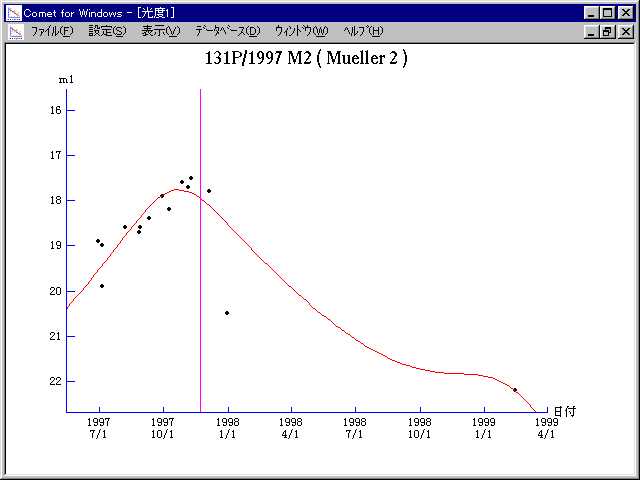# \$B%_%e%i!

131P/Mueller 2 (1997)###\$B%W%m%U%#!<%k(B

 \$BId9f(B 131P/1997 M2 \$B8!=PF|(B 1997\$BG/(B6\$B7n(B29\$BF|(B, 7\$B7n(B5\$BF|(B \$B8!=P8wEY(B 18.9\$BEy(B, 19.9\$BEy(B \$B8!=P \$B?y9>=_(B (\$B%@%\$%K%C%/E7J8Bf(B), \$BCfB<>4@5(B (\$B5WK|9b86(B)

###\$B50F;MWAG(B

```   The following improved orbital elements by Kenji Muraoka, are
from 147 observations 1990 Sept. 15 to 1999 Feb. 14, perturbations
by 9 Plantes, Moon and 5 minor planets were taken into account.
The mean residual is +/- 0.83 arc seconds.

Epoch  =  1997 Nov.  8.0  TT       JDT = 2450760.5
T  =  1997 Nov. 22.20835       +/- 0.00037 (m.e.) TT
Peri. =  179.64060                +/- 0.00021
Node  =  214.28496                +/- 0.00020   (2000.0)
Incl. =    7.35544                +/- 0.00005
q  =    2.4121430              +/- 0.0000034 AU
e  =    0.3438991              +/- 0.0000010
a  =    3.6764819              +/- 0.0000020 AU
n  =    0.13981553             +/- 0.00000012
P  =    7.049                  +/- 0.0000059  years
```

###\$B@1?^(B1996\$BG/(B 6\$B7n(B 6\$BF|!A(B1998\$BG/(B 3\$B7n(B 8\$BF|(B1997\$BG/(B 7\$B7n(B11\$BF|!A(B1999\$BG/(B 5\$B7n(B22\$BF|(B

###\$B8wEYJQ2=(B

```        m1 = 9.3 + 5 log\$B&\$(B + 20.0 log r
```##### \$B50F;MWAG\$OB<2,7r<#;a\$N7W;;\$K\$h\$k\$b\$N\$G\$9!#(B \$B@1?^\$O(B StellaNavigator Ver.2.0 for Windows (\$B%"%9%H%m%"!<%D(B \$BJTCx(B / \$B%"%9%-!<=PHG6I4)(B) \$B\$G:n@.\$7\$?\$b\$N\$G\$9!#(B \$B8wEY%0%i%U\$O(BComet for Windows\$B\$G:n@.\$7\$?\$b\$N\$G\$9!#(B